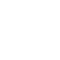# Custom Angled Triangle Maker Script for AI

Today I would like to share with you a great handy script by Carlos Canto. Custom Angled Triangle Maker for Adobe Illustrator…

Recently I was on a project about patterns, I was searching and found one to try on Illustrator. Then I have noticed that we don’t have an easy solution for creating custom angled triangle. I could not believe that powerful Illustrator does not have that feature. I have looked around net but I could not find any practical solution. The closest tutorial I have found was (link) containing too many steps. Since I was going to make lots of various patterns that was not a good solution for me. I had needed a script and posted my question to Adobe Illustrator Script Forum. Thankfully Carlos Canto answered my question and wrote this whole great scriptThere are two varieties for this script. One is Side, Side, Angle and the other one is Side,Angle,Angle.

[divider]

// Triangle Maker (Side, Side, Angle)

// by Carlos Canto – 11/21/13; // Shared by Creativetuts.com

var idoc = app.activeDocument; dw = idoc.width; dh = idoc.height; wc= dw /2; hc = dh *-0.5;

var s_ssa = Window.prompt (‘Enter 2 Sides in points, Angle in degrees, (Side, Side, Angle)’, ‘100, 120, 30’, ‘Triangle Maker’); var a_ssa = s_ssa.split(‘,’); // turn into an array

var side1 = parseFloat (a_ssa); var side2 = parseFloat (a_ssa); var angle = parseFloat (a_ssa);

var path1 = idoc.pathItems.add(); var p0 = [0,0]; var p1 = [side1,0]; var p2 = getSideAngePoint (side2, angle);

path1.setEntirePath ([p0, p1, p2]); path1.closed = true; path1.position = Array(wc,hc);

// returns point 2 given a segment length and its angle, point 1 is [0,0] function getSideAngePoint(side, angle) { var rads = angle*Math.PI/180; var px = Math.cos(rads)*side; var py = Math.sin(rads)*side;

return [px, py]; }

[divider]

// Triangle Maker (Side, Angle, Angle)
// by Carlos Canto – 11/21/13;
// Shared by Creativetuts.com

var idoc = app.activeDocument;
dw = idoc.width;
dh = idoc.height;
wc= dw /2;
hc = dh *-0.5;

var s_ssa = Window.prompt (‘Enter 1 Side in points, 2 Angles in degrees, (Side, Angle1, Angle2)’, ‘120, 30, 57’, ‘Triangle Maker’);
var a_ssa = s_ssa.split(‘,’); // turn into an array

var side = parseFloat (a_ssa);
var angle1 = parseFloat (a_ssa);
var angle2 = parseFloat (a_ssa);

if (angle1+angle2<180) {
var p0 = [0,0];
var p1 = getSideAngePoint (side, angle1);

var C = get3rdAngle (angle1, angle2);
var teta = getTempAngle (C); // the one that forms a Right Angle Triangle, down from Side Point 2 and hipotenuse
var opo = oposite (adj, teta);

var p2x = p1+opo;

var p2 = [p2x,0];

path1.setEntirePath ([p0, p1, p2]);
path1.closed = true;
path1.position = Array(wc,hc);
}
else {alert(‘both angles combined should be less than 180’);}

// returns point 2 given a segment length and its angle, point 1 is [0,0]
function getSideAngePoint(side, angle) {

return [px, py];
}

// given 2 angles, return the 3rd angle
function get3rdAngle(angle1, angle2) {
return 180-angle1-angle2;
}

// return “TETA” in a complimentary Right Angle Triangle
function getTempAngle(thirdAngle) {
return 90-thirdAngle;
}

// returns the Oposite side in the complimentary Right Angle Triangle
return op;
}

[divider]

[wpdm_file id=31 title=”true” ]

[wpdm_file id=32 title=”true” ]

1.Du pont says:

whoah this blog is great i love studying your articles.

Keep up the good work! You realize, many persons are looking round
for this information, you can help them greatly.